# Intelligent AI? Here's How You Can Evaluate.

##### 3 intriguing tests to measure the IQ of your neural nets.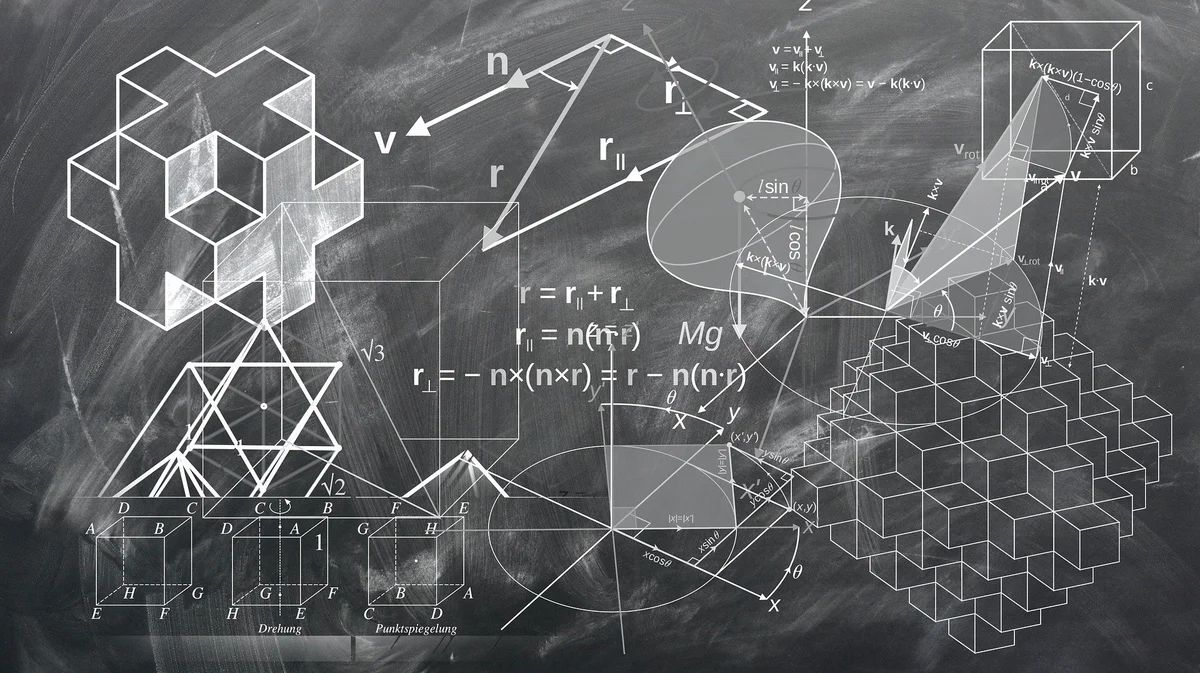One of the long-standing goals of artificial intelligence is to develop machines with abstract reasoning capabilities equal to or better than humans. Though there has also been substantial progress in both reasoning and learning in neural networks, the extent to which these models exhibit anything like general abstract reasoning is the subject of much debate.

Neural networks have perfected the technique to identify cats in images and translating from one language to another. Is that intelligence or they are just great at memorizing? How can we measure the intelligence of neural networks?

Some researchers have been developing ways to evaluate neural networks’ intelligence. It’s not using mean squared error or entropy loss. But they are giving neural networks an IQ test, high school mathematics questions, and comprehension problems.

# Pattern Matching

A human’s capacity for abstract reasoning can be estimated using a visual IQ test developed by psychologist John Raven in 1936: the Raven’s Progressive Matrices (RPMs). The premise behind RPMs is simple: one must reason about the relationships between perceptually obvious visual features, such as shape positions or line colors, and choose an image that completes the matrix.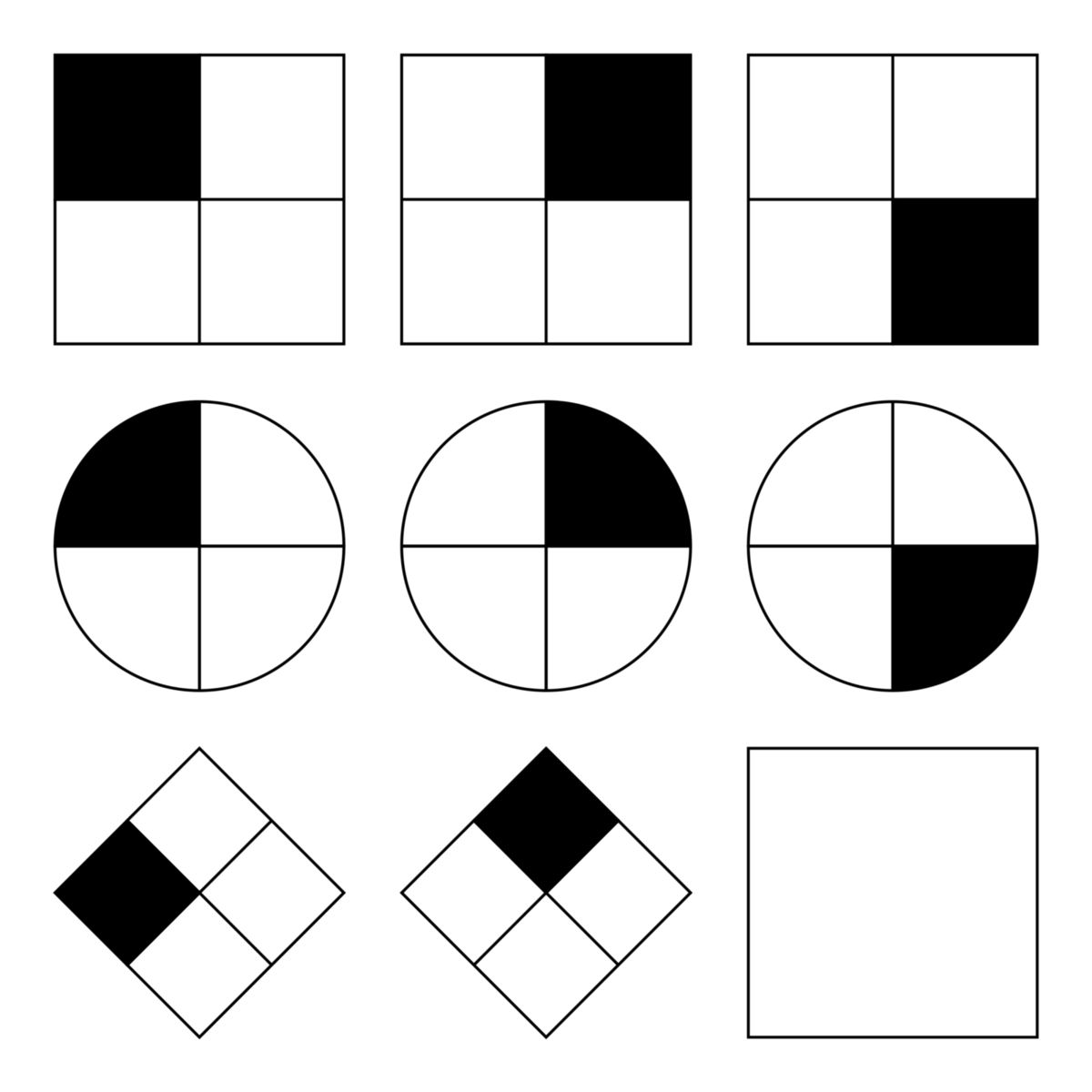Since one of the goals of AI is to develop machines with similar abstract reasoning capabilities to humans, researchers at Deepmind proposed an IQ test for AI, designed to probe their abstract visual reasoning ability. In order to succeed in this challenge, models must be able to generalize well for every question.In this study, they compared the performance of several standard deep neural networks and proposed two models that include modules that specially designed for abstract reasoning:

• standard CNN-MLP: (four-layers convolutional neural network with batch normalization and ReLU)
• ResNet-50: as described in He et al. (2016)
• LSTM: 4 layers CNN followed by LSTM
• Wild Relation Network (WReN): answers are selected and evaluated using a Relation Network designed for relational reasoning
• Wild-ResNet: variant of the ResNet that is designed to provide a score for each answer
• Context-Blind ResNet: ResNet-50 model with eight multiple-choice panels as input without considering the context

The IQ test questions aren’t challenging enough; so they added various shapes, lines of varying thickness, and colors, as distractions.The best performing model is the WReN model! This is due to the Relation Network module designed explicitly for reasoning about the relations between objects. After removing distractions, the WReN model performed notably better at 78.3%, compared with 62.6% with distractions!# Mathematical Reasoning

Mathematical reasoning is one of the core abilities of human intelligence. Mathematics presents some unique challenges as humans do not primarily understand and solve mathematical problems based on experiences. Mathematical reasoning is also based on the ability to infer, learn, and follow symbol manipulation rules.

Researchers are Deepmind released a dataset consisting of 2 million mathematics questions. These questions are designed for neural networks for measuring mathematical reasoning. Each question is limited to 160 characters in length, and answers to 30 characters. The topics include:

• algebra (linear equations, polynomial roots, sequences)
• arithmetic (pairwise operations and mixed expressions, surds)
• calculus (differentiation)
• comparison (closest numbers, pairwise comparisons, sorting)
• measurement (conversion, working with time)
• numbers (base conversion, remainders, common divisors and multiples, primality, place value, rounding numbers)
• polynomials (addition, simplification, composition, evaluating, expansion)
• probability (sampling without replacement)The dataset comes with two sets of tests:

• interpolation (normal difficulty): a diverse set of question types
• extrapolation (crazy mode): difficulty beyond those seen during training dataset, with problems involving larger numbers, more numbers, more compositions, and larger samplers

In their study, they investigated a simple LSTM model, Attentional LSTM, and Transformer. Attentional LSTM and Transformer architectures parse the question with an encoder and the decoder will produce the predicted answers one character at a time.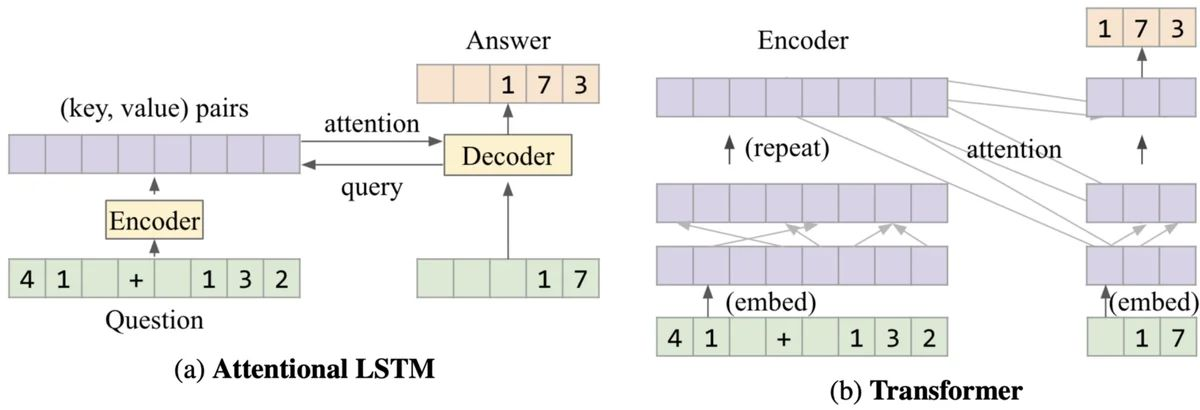They also replaced LSTM with relational memory core, which consists of multiple memory slots that interact via attention. In theory, these memory slots seem useful for mathematical reasoning as models can learn to use the slots to store mathematical entities.This is what they have found:

• The relational memory core did not help in performance. They reasoned that perhaps it is challenging to learn to use slots for manipulating mathematical entities.
• Both simple LSTM and Attentional LSTM have similar performance. Perhaps the attention modules are not learning to parse the question algorithmically.
• The Transformer outperforms other models as perhaps more similar to sequential reasoning of how a human solve mathematical problems.

# Language Understanding

In recent years, there has been notable progress across many natural language processing methods, such as ELMo, OpenAI GPT, and BERT.

Researchers introduced the General Language Understanding Evaluation (GLUE) benchmark in 2019, designed to evaluate the performance of models across a nine English sentence understanding tasks, such as question answering, sentiment analysis, and textual entailment. These questions cover a broad range of domains (genres) and difficulties.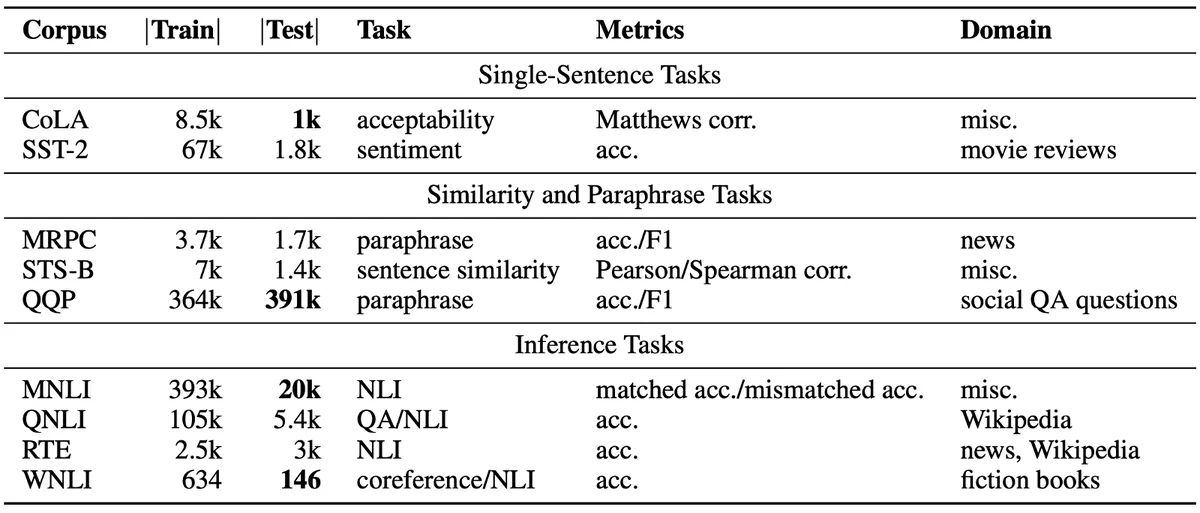With human performance at 1.0, these are the GLUE score for each language model. Within the same year that GLUE was introduced, researchers have developed methods that surpass human performance. It seems like GLUE is too easy for neural networks; thus, this benchmark is no longer suitable.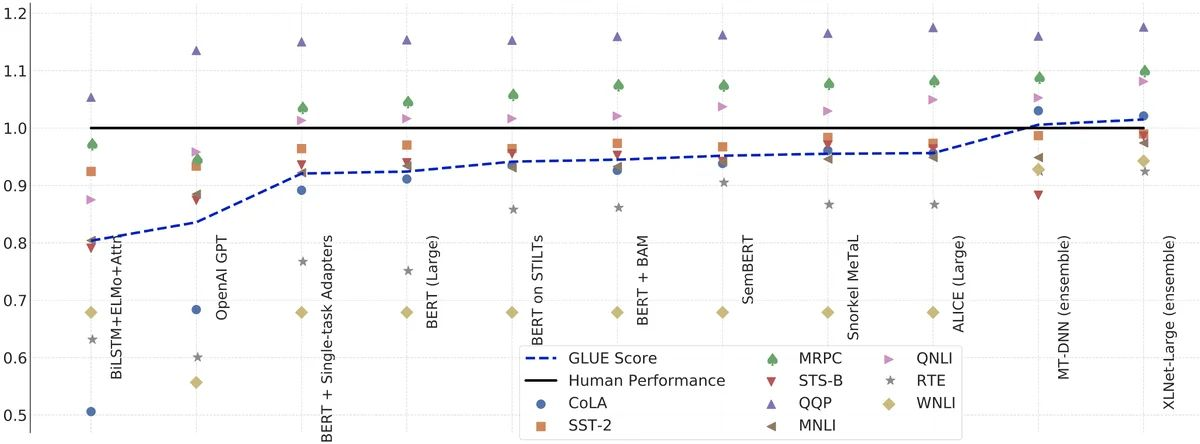SuperGLUE was introduced as a new benchmark designed to pose a more rigorous test of language understanding. The motivation of SuperGLUE is the same as GLUE, to provide a hard-to-game measure of progress toward general-purpose language understanding technologies for the English language.Researchers have evaluated the BERT-based models and find that they still lag behind humans by nearly 20 points. Given the difficulty of SuperGLUE, further progress in multi-task, transfer, and unsupervised/self-supervised learning techniques will be necessary to approach human-level performance on the benchmark.

Let us see how long it takes before machine learning models surpass human capability again, such that new tests have to be developed.